## Standard Map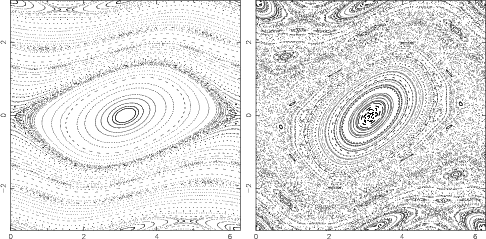A 2-D Map, also called the Taylor-Greene-Chirikov Map in some of the older literature.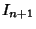(1)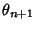(2)

whereandare computed mod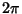and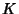is a Positive constant. An analytic estimate of the width of the Chaotic zone (Chirikov 1979) finds(3)

Numerical experiments give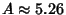and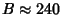. The value ofat which global Chaos occurs has been bounded by various authors. Greene's Method is the most accurate method so far devised.

 Author Bound Fraction Decimal Hermann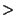0.029411764 Italians- 0.65 Greene- 0.971635406 MacKay and Pearson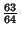0.984375000 Mather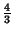1.333333333

Fixed Points are found by requiring that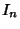(4)(5)

The first gives, so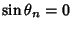and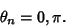(6)

The second requirement gives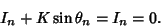(7)

The Fixed Points are thereforeand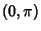. In order to perform a Linear Stability analysis, take differentials of the variables(8)(9)

In Matrix form,(10)

The Eigenvalues are found by solving the Characteristic Equation(11)

so(12)(13)

For the Fixed Point,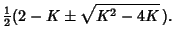(14)

The Fixed Point will be stable ifHere, that means(15)(16)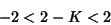(17)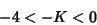(18)

so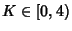. For the Fixed Point (0, 0), the Eigenvalues are(19)

If the map is unstable for the larger Eigenvalue, it is unstable. Therefore, examine. We have(20)

so(21)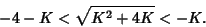(22)

But, so the second part of the inequality cannot be true. Therefore, the map is unstable at the Fixed Point (0, 0).

References

Chirikov, B. V. A Universal Instability of Many-Dimensional Oscillator Systems.'' Phys. Rep. 52, 264-379, 1979.